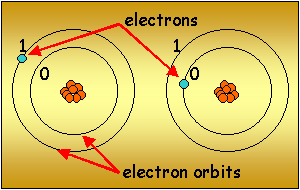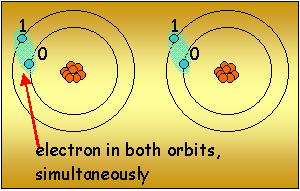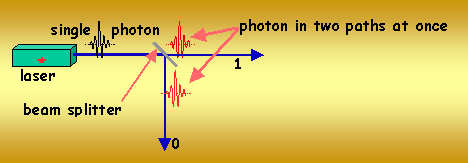## Contents

### Moore's Law and the future of computers

In 1965 Intel co-founder Gordan Moore noted that processing power (number of transistors and speed) of computer chips was doubling each 18 months or so. This trend has continued for nearly 4 decades. But can it continue? The basic processing unit in a computer chip is the transistor which acts like a small switch. The binary digits 0 and 1 are represented by the transistor being turned off or on.Currently thousands of electrons are used to drive each transistor. As the processing power increases, the size of each transistor reduces. If Moore's law continues unabated, then each transistor is predicted to be as small as a hydrogen atom by about 2030, as illustrated in the graph. At that size the quantum nature of electrons in the atoms becomes significant and generates errors in the computation.

However, rather than be a hindrance, it is possible to exploit the quantum physics as a new way to do computation. And this new way opens up fantastic new computational power based on the wave nature of quantum particles.

### Particle-wave duality

We normally think of electrons, atoms and molecules as particles.  But each of these objects can also behave as waves. This dual particle-wave behaviour was first suggested in the 1920's by Louis de Broglie.

This concept emerged as follows.  Thomas Young's experiments with double slits in the early 1800's shows that light behaves as if it is a wave. But, strikingly, Einstein's explanation of the photoelectric effect in 1905 shows that light consists of particles. In 1923 de Broglie suggested this dual particle-wave property might apply to all particles including electrons. Then in 1926 Davisson and Germer found that electrons scattered off a crystal of nickel behaved as if they were waves. Since then neutrons, atoms and even molecules (including bucky balls) have been shown to behave as waves.  The waves tell us where the particle is likely to be found.

This dual particle-wave property is exploited in quantum computing in the following way. A wave is spread out in space. In particular, a wave can spread out over two different places at once. This means that a particle can also exist at two places at once. This concept is called the superposition principle - the particle can be in a superposition of two places.

### Bits and QubitsThe basic data unit in a conventional (or classical) computer is the bit, or binary digit. A bit stores a numerical value of either 0 or 1. An example of how bits are stored is given by a CD rom: "pits" and "lands" (absence of a pit) are used to store the binary data.

We could also represent a bit using two different electron orbits in a single atom.  In most atoms there are many electrons in many orbits.  But we need only consider the orbits available to a single outermost electron in each atom.  The figure on the right shows two atoms representing the binary number 10.  The inner orbits represent the number 0 and the outer orbits represent the binary number 1.  The position of the electron gives the number stored by the atom.However, a completely new possibility opens up for atoms. Electrons have a wave property which allows a single electron to be in two orbits simultaneously.  In other words, the electron can be in a superposition of both orbits.  The figure on the left shows two atoms each with a single electron in a superposition of two orbits.  Each atom represents the binary numbers 0 and 1 simultaneously.  The two atoms together represent the 4 binary numbers 00, 01, 10 and 11 simultaneously.

To distinguish this new kind data storage from a conventional bit, it is called a quantum bit which is shortened to qubit.  Each atom in the figure above is a qubit.  The key point is that a qubit can be in a superposition of the two numbers 0 and 1. Superposition states allow many computations to be performed simultaneously, and gives rise to what is known as quantum parallelism

Another example of a qubit is a photon (a particle of light) travelling along two possible paths.  Consider what happens when a photon encounters a beam splitter.   A beam splitter is just like an ordinary mirror, however the reflective coating is made so thin that not all light is reflected and some light is transmitted through the mirror as well.  When a single photon encounters a beam splitter, the photon emerges in a superposition of the reflected path and the transmitted path.  One path is taken to be the binary number 0, and the other path is taken to be the number 1.  The photon in a superposition of both paths and so represents both 0 and 1 simultaneously.A simulator of a quantum computer based on this idea of a photon being in many paths is given in the section Simulator.  Many quantum systems can be used as qubits.   More details are given in the section Qubit Systems.

### Quantum parallelism

A one bit memory can store one of the numbers 0 and 1.  Likewise a two bit memory can store one of the binary numbers 00, 01, 10 and 11 (i.e. 0, 1, 2 and 3 in base ten).  But these memories can only store a single number (e.g. the binary number 10) at a time.

As described above, a quantum superposition state allows a qubit to store 0 and 1 simultaneously.  Two qubits can store all the 4 binary numbers 00, 01, 10 and 11 simultaneously.  Three qubits stores the 8 binary numbers 000, 001, 010, 011, 100, 101, 110 and 111 simultaneously.   The table below shows that 300 qubits can store more than 1090 numbers simultaneously.  That's more than the number of atoms in the visible universe!

This shows the power of quantum computers:  just 300 photons (or 300 ions etc.) can store 2300~1090 numbers simultaneously. This is more numbers than there are atoms in the universe, and calculations can be performed simultaneously on each of these numbers!

 qubits stores simultaneously total number 1 (0 and 1) 21 = 2 2 (0 and 1)(0 and 1) 2x2 = 22 = 4 3 (0 and 1)(0 and 1)(0 and 1) 2x2x2 = 23 = 8 : : : 300 (0 and 1)(0 and 1)........(0 and 1) 2x2......x2 = 2300